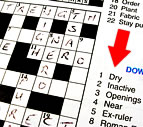×

divide

5 ENTRIES FOUND:
1 divide /dəˈvaɪd/ verb
divides; divided; dividing
1 divide
/dəˈvaɪd/
verb
divides; divided; dividing
Learner's definition of DIVIDE
: to separate (something) into two or more parts or pieces
[+ object]
often used as (be) divided
[no object]
[+ object] : to separate (something) into classes or categories
often used as (be) divided
[+ object]
: to give (something) out in usually equal amounts
: to use (your time, energy, etc.) for two or more purposes or activities usually + between
[+ object] : to cause (something) to be separate or apart from something else
often + from
[+ object]
: to separate (people) into groups that disagree
often used as (be) divided
: to cause (opinions, views, etc.) to not agree usually used as (be) divided
mathematics : to calculate how many times one number contains another number
[+ object]
[no object]
compare multiply

divide and conquer

or divide and rule
: to make a group of people disagree and fight with one another so that they will not join together against you
2 divide /dəˈvaɪd/ noun
plural divides
2 divide
/dəˈvaɪd/
noun
plural divides
Learner's definition of DIVIDE
[count]
chiefly US : a line of hills or mountains from which rivers drain : a ridge between two rivers :
: a separation of people into two or more groups that is caused by different opinions or beliefs or by a disagreement usually singular
usually + among or between